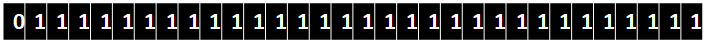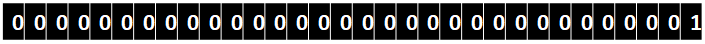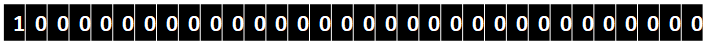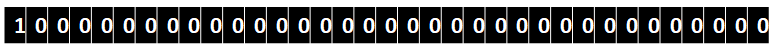GFG App
Open AppBrowser
Continue

# Overflow of Values While Typecasting in C ++

C++ datatypes have a specific size, that is, the number of bits that each of them occupies is fixed. Sometimes while writing code, we may end up assigning a value that is greater than the maximum capacity of the datatype to store. In that case, an overflow occurs, and depending on the datatype the compiler handles it differently.

## Overflow of Integer Values

If we try to typecast a number that is greater than INT32_MAX or less than INT32_MIN, overflow occurs, in that case, the compiler first calculates the value of the given expression and then stores the last possible bits inside the datatype and then manipulates it.

Example:

## C++

 `#include ` `using` `namespace` `std; ` ` `  `int` `main() { ` ` `  `    ``cout << ``int``(INT32_MAX + 1) << endl; ` `    ``return` `0; ` `} `

Output

`-2147483648`

Here we were adding INT32_MAX with 1, so by binary addition, it goes something like this.INT32_MAX1INT32_MIN

Explanation: So, first, the compiler adds INT32_MAX with 1 and comes up with a binary equivalent number, but this is beyond the range of int because the first bit was supposed to be reserved for denoting the sign, but as we typecast it, this number is forced to be an integer and its last 32 bits are stored as an int, and when we print this number, the compiler takes the first bit as a sign bit and finds the 2’s complement of the number and then print it.

Example:

## C++

 `#include ` `using` `namespace` `std; ` ` `  `int` `main() { ` ` `  `    ``cout << ``int``(17179869184) << endl; ``// pow(2, 34) ` `    ``return` `0; ` `} `

Output

`0`

Let’s understand what happened here too. The Binary representation of pow(2, 34) is :When we try to typecast it to a 32-bit int, then it can only store 32 bits at max, so the compiler converts pow(2, 34) to binary and stores the last 32 bits as an integer, as we can clearly see that the last 32 bits are all 0, therefore the resultant number is zero.

## Overflow of Boolean Values

For the bool datatype, any number except for zero is treated as true, so if we typecast a number other than 0, it will store 1.

Example:

## C++

 `#include ` `using` `namespace` `std; ` ` `  `int` `main() { ` ` `  `    ``cout << ``bool``(4) << endl; ` `      ``cout << ``bool``(0) << endl; ` `      ``cout << ``bool``(INT32_MIN) << endl; ` `    ``return` `0; ` `} `

Output

```1
0
1```

## Overflow of char Values

The compiler distinguishes whether a stream of 4 bytes is a single integer or 4 different characters by referring to the datatype of the variable. However, when we are converting one datatype to another, the value might be too big for the second datatype to hold, in that case, an overflow of values occurs.

Example:

## C++

 `// C++ ` `#include ` `using` `namespace` `std; ` ` `  `int` `main() ` `{ ` `    ``// null, because char values ranges from 0 ` `    ``// to 255 and upon entering 256 (100000000), only the ` `    ``// last byte (8 bits) of ` `    ``//  binary form of 256(00000000) is stored ` `    ``char` `a = 256; ` `    ``cout << a << endl; ` `    ``return` `0; ` `}`

Output:

` `

But we can typecast it to character explicitly. In that case, the value stored in the char will be (num % 256).

(0 to 255) comprises 256 numbers so the value stored will be (number % 256)

My Personal Notes arrow_drop_up